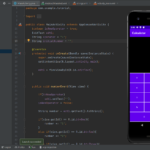## Calculator App In Android With Source Code

The Calculator app is a simple Android project. It functions fully as similar to a normal calculator. Here you can perform all of your calculations with ease. To run the project you will need Android Studio. So before you run the project make sure that yo...

Type : Project

File Size : 11.0 MB

Please click the button below to download the ZIP File.# Similar Projects and Reports

#### Calculator App In Android With Source Code

The Calculator app is a simple Android project. It functions fully as similar to a normal calculator. Here you can perform all of your calculations with ease. To run the project you will need Android Studio. So before you run the project make sure that yo...

#### Calculator App In C# With Source Code

This Calculator App in C# is based on the concept of normal mathematical operations on the calculator. The project contains the numbers, operators, and signs like in a normal calculator. Here, the user cannot enter the number themselves, they can just cli...

#### Simple Calculator In Android With Source Code

The Simple Calculator is a simple beginners level Android project. It functions fully as similar to a normal calculator. Here you can perform all of your calculations with ease. To run the project you will need Android Studio. So before you run the projec...

#### Voice Calculator App In C# With Source Code

Voice Calculator App in C# is based on the concept of voice-over mathematical operations on the calculator. The project contains the numbers, operators, and signs like in a normal calculator. Here, the user can also enter the number themselves for the cal...

#### CALCULATOR IN C++ WITH SOURCE CODE

This project is based on a concept to generate the total sum, subtractions, divisions and multiplication. User has to input numbers with mathematical signs (“+,-,*,/”), the system will display the output. [ user has to input : 5*5, then the system will d...

#### Calculator App In Python With Source Code

The Calculator App In Python is a simple project developed using Python. The project contains the numbers, operators, and signs like on a normal calculator. So, the user cannot enter the number themselves, they can just click on the numbers they want in t...

Visit code-projects.org to download thousands of other free projects